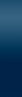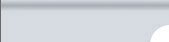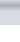MenuToolbarConstraints/ResultsImpedance SpectraEquivalent CircuitSettings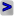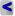EIS Spectrum Analyser Help User-Defined Element (U-element) Use the U-element only when the expected dependence cannot be represented with the predefined elements, the predefined elements work much faster. 1. Add an U-element to the Equivalent Circuit panel and click the U-element page control in Settings panel. 2. Select the number of parameters required in the model (up to five parameters in Beta version).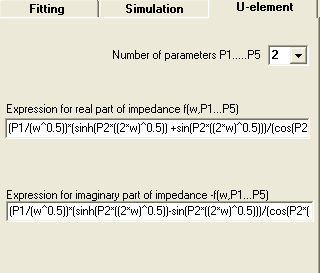3. Define the U-element impedance dependence on frequency: type an expression for real part of impedance into the ReZ box and the corresponding expression for the negative of imaginary part of impedance into the ImZ box (omit the imaginary unit). Use “w” for the circular frequency, P1, P2… for parameters. The following operators and functions are allowed: * Multiplication + Addition /  Division - Subtraction, negative value ^ Exponentiation cos()  for the cosine cotnh()  for the hyperbolic cotangent sin()  for the sine tan()  for the tangent cotn()  for the cotangent arctn()  for the arctangent cosh()  for the hyperbolic cosine arcsn()  for the arcsine sinh()  for the hyperbolic sine arccn()  for the arccosine tanh()  for the hyperbolic tangent ln()  for the natural logarithm log()  for the common logarithm exp()  for the exponent use pi for π-constant Use round brackets (nested round brackets also may be used) to define sequence of operations. Use the decimal point to enter non-integral numbers. “E” may be used for a power of 10, e.g. 2E-5. Example. Capacitor has no real impedance and has -1/(w*C) imaginary impedance. To determine an U-element equivalent to the capacitor, select 1 for the number of parameters, type 0 in the ReZ box (expression for real part of impedance), type 1/(w*P1) in the ImZ box (expression for imaginary part of impedance, “-” omitted). Two more examples: CPE as U-element: Re[Z] = (1/(P1*w^P2))*cos(P2*pi) Im[Z] = (1/(P1*w^P2))*sin(P2*pi) Warburg short (Ws) as U-element: Re[Z] = (P1/(w^0.5))*(sinh(P2*((2*w)^0.5)) +sin(P2*((2*w)^0.5)))/(cos(P2*((2*w)^0.5)) + cosh(P2*((2*w)^0.5))) Im[Z] = (P1/(w^0.5))*(sinh(P2*((2*w)^0.5)) - sin(P2*((2*w)^0.5)))/(cos(P2*((2*w)^0.5)) + cosh(P2*((2*w)^0.5))) EIS Spectrum Analyser, 2008